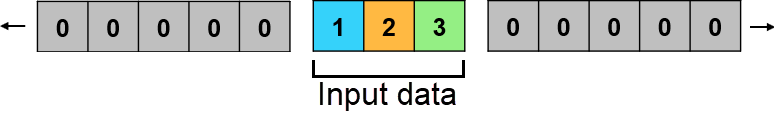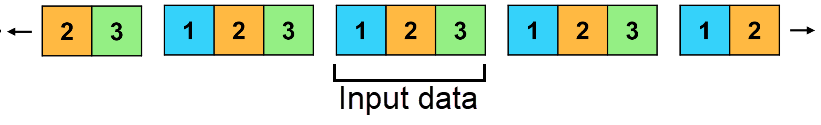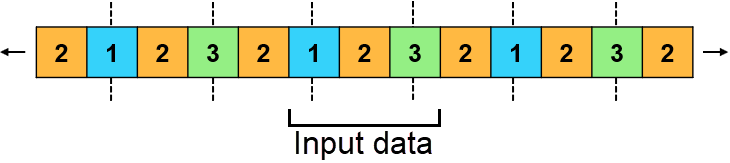Since R2023b

## Syntax

``B = paddata(A,m)``
``B = paddata(A,m,Name=Value)``

## Description

example

````B = paddata(A,m)` pads `A` to size `m` by adding elements to the trailing side of `A`. For example, for a scalar size `m`: If `A` is a vector, then `paddata(A,m)` pads `A` to length `m`.If `A` is a matrix, table, or timetable, then `paddata(A,m)` pads `A` to have `m` rows.If `A` is a multidimensional array, then `paddata(A,m)` pads `A` to the size specified by `m` along the first dimension whose size is greater than 1. If `m` is less than or equal to the size of `A` in the operating dimension, then `paddata` returns all of `A`.```

example

````B = paddata(A,m,Name=Value)` specifies additional parameters for padding using one or more name-value arguments. For example, `paddata(A,m,Pattern="circular")` pads by repeating the input data circularly.```

## Examples

collapse all

Create a four-element column vector, and pad the vector to six elements. By default, the `paddata` function adds zeros to the trailing size of the numeric vector.

```A = [1; 3; 5; 7]; B = paddata(A,6)```
```B = 6×1 1 3 5 7 0 0 ```

Pad a row vector to six elements.

```A2 = [2 4 6 8]; B2 = paddata(A2,6)```
```B2 = 1×6 2 4 6 8 0 0 ```

Create two vectors with different lengths.

```A1 = [2; 8; 3; 5]; A2 = [9; 4; 6; 2; 7; 7; 0];```

Determine the length of the longer vector.

```szA1 = size(A1,1); szA2 = size(A2,1); [m,idx] = max([szA1 szA2])```
```m = 7 ```
```idx = 2 ```

Pad the shorter vector to match the length of the longer vector.

`B1 = paddata(A1,m)`
```B1 = 7×1 2 8 3 5 0 0 0 ```

You can concatenate vectors of the same length. Create a matrix using the two vectors.

`C = [B1 A2]`
```C = 7×2 2 9 8 4 3 6 5 2 0 7 0 7 0 0 ```

Create a 3-by-3 matrix. Pad the columns to a length of 4 by adding one element to each column. Pad the rows to a length of 6 by adding three elements to each row.

`A = [1 3 5; 2 4 6; 7 8 10]`
```A = 3×3 1 3 5 2 4 6 7 8 10 ```
`B = paddata(A,[4 6])`
```B = 4×6 1 3 5 0 0 0 2 4 6 0 0 0 7 8 10 0 0 0 0 0 0 0 0 0 ```

Create a 3-by-3 matrix as the first page in a 3-D array. Add a second page to the array by padding along the third dimension.

`A = [1 3 5; 2 4 6; 7 8 10]`
```A = 3×3 1 3 5 2 4 6 7 8 10 ```
`B = paddata(A,2,Dimension=3)`
```B = B(:,:,1) = 1 3 5 2 4 6 7 8 10 B(:,:,2) = 0 0 0 0 0 0 0 0 0 ```

Create a timetable with variables of different data types.

```num = [10; 20; 30]; cat = categorical(["A"; "B"; "A"]); log = logical([1; 0; 1]); str = ["Text 1"; "Text 2"; "Text 3"]; TT = timetable(num,cat,log,str,Timestep=hours(2))```
```TT=3×4 timetable Time num cat log str ____ ___ ___ _____ ________ 0 hr 10 A true "Text 1" 2 hr 20 B false "Text 2" 4 hr 30 A true "Text 3" ```

Pad each timetable variable. `paddata` uses the default fill value for the data type of each variable. The default fill value for each data type is the same as the value of elements that MATLAB® creates when assigning a value beyond the last row of the table.

`B1 = paddata(TT,6)`
```B1=6×4 timetable Time num cat log str _____ ___ ___________ _____ _________ 0 hr 10 A true "Text 1" 2 hr 20 B false "Text 2" 4 hr 30 A true "Text 3" 6 hr 0 <undefined> false <missing> 8 hr 0 <undefined> false <missing> 10 hr 0 <undefined> false <missing> ```

Specify a custom fill value for the elements to add to each timetable variable. `paddata` extends the row times, so you do not need to specify a fill value for the row times.

`B2 = paddata(TT,6,FillValue={mean(num),"C",1,""})`
```B2=6×4 timetable Time num cat log str _____ ___ ___ _____ ________ 0 hr 10 A true "Text 1" 2 hr 20 B false "Text 2" 4 hr 30 A true "Text 3" 6 hr 20 C true "" 8 hr 20 C true "" 10 hr 20 C true "" ```

Create a column vector, and pad the vector by repeating the leading element.

```A = [1; 3; 5; 7]; B = paddata(A,7,Pattern="edge",Side="leading")```
```B = 7×1 1 1 1 1 3 5 7 ```

## Input Arguments

collapse all

Input data, specified as a vector, matrix, multidimensional array, table, timetable, cell array, or structure array.

Note

If `A` is a cell array, then `paddata` changes the size of the entire array. It does not change the size of each cell in the array. Use the `cellfun` function to apply `paddata` to each cell in a cell array.

Size of padded data along operating dimension, specified as a nonnegative integer scalar or vector of nonnegative integers. Each element represents the size of the padded data in an operating dimension.

• If `m` is greater than the size of `A` in the operating dimension, then `paddata` adds elements.

• If `m` is less than or equal to the size of `A` in the operating dimension, then `paddata` returns the input data without adding elements.

### Name-Value Arguments

Specify optional pairs of arguments as `Name1=Value1,...,NameN=ValueN`, where `Name` is the argument name and `Value` is the corresponding value. Name-value arguments must appear after other arguments, but the order of the pairs does not matter.

Example: `B = paddata(A,m,Pattern="circular")`

Dimensions to operate along, specified as `"auto"`, a positive integer scalar, or a vector of positive integers. Each element represents a dimension of the input data.

If `Dimension` is `"auto"`, then the operating dimension depends on the input arguments:

• If `m` is a scalar and `A` is an array, then the operating dimension is the first dimension whose size is greater than 1.

• If `m` is a vector, then the operating dimensions are `1:numel(m)`.

• If `A` is a table or timetable, then the operating dimension is `1`, and operation is along each table or timetable variable separately.

Fill value for added elements, specified as a scalar, cell array, or scalar structure.

The default fill value for each class is the same as the value of elements that MATLAB® creates when assigning a value past the end of a vector. For example, the default fill value for numeric input data is 0.

• If `A` is an array, then a scalar `FillValue` indicates the value for all elements added to `A` during padding.

• If `A` is a table or timetable, then a cell array `FillValue` indicates a different fill value for elements added to each table or timetable variable. The number of cells in the cell array must match the number of table or timetable variables. If `A` is a table with row names, then `paddata` extends the row names with the default row name, such as `RowN`; it does not pad the row names using `FillValue`. If `A` is a timetable with row times, then `paddata` extends the row times; it does not pad the row times using `FillValue`.

• If `A` is a structure array, then a scalar structure `FillValue` indicates a different fill value for each field in the input data. The number and names of fields in `FillValue` must match the number and names of fields in the input data.

If you specify `FillValue`, you cannot specify `Pattern`.

Pattern for adding elements, specified as one of the pattern names in the table. The pattern is repeated until the resized data has size `m`.

• If `A` is a table with row names, then `paddata` extends the row names with the default row name, such as `RowN`; it does not add to the row names using `Pattern`.

• If `A` is a timetable with row times, then `paddata` extends the row times; it does not add to the row times using `Pattern`.

If you specify `Pattern`, you cannot specify `FillValue`.

This table lists the pattern names with a description and a sample of how each pattern pads the input data `A = [1 2 3]`.

`"constant"`Pad data with the default value determined by the data type of `A`.`"edge"`Pad data by replicating the leading and trailing endpoints as constant fill values.`"circular"`Pad data by repeating the input data circularly.`"flip"`Pad data by flipping the input data symmetrically. Endpoints are duplicated.`"reflect"`Pad data by reflecting the input data. Endpoints are not duplicated.Side of input data for padding, specified as one of these values:

• `"trailing"` — Pad `A` with trailing elements.

• `"leading"` — Pad `A` with leading elements.

• `"both"` — Pad `A` on both sides. If the number of elements to add in the operating dimension is even, then pad the trailing and leading sides of `A` evenly. If the number of elements to add in the operating dimension is odd, then pad the remaining element on the trailing side of `A`.

## Tips

• `paddata` only adds elements to the input data. `paddata` is recommended if you do not want to remove elements from your data and you do not require the resized data to match the target size. If you require the resized data to respect the sizes in `m`, then consider using the `resize` function, which can also remove elements from your data.

## Version History

Introduced in R2023b